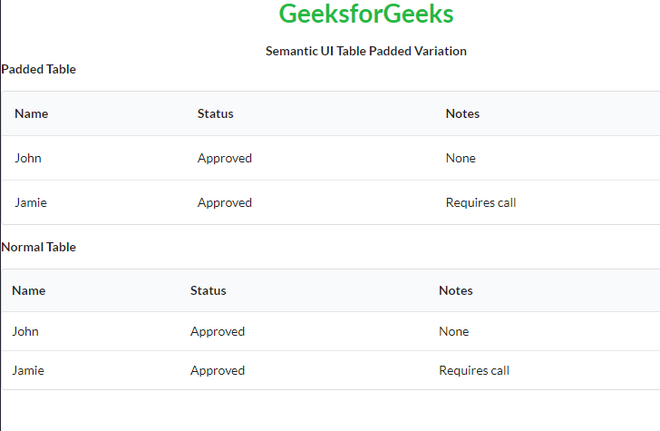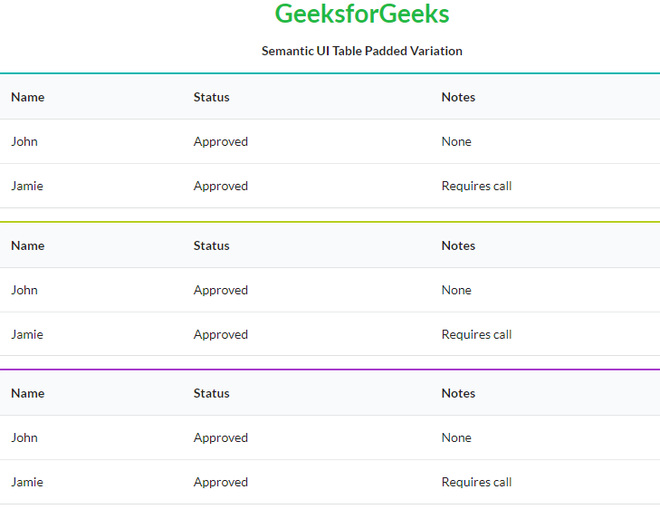GFG App
Open AppBrowser
Continue

Semantic UI is an open-source framework that uses CSS and jQuery to build great user interfaces. It is the same as a bootstrap for use and has great different elements to use to make your website look more amazing. It uses a class to add CSS to the elements.

Tables are an easy way to organize a lot of data. A table is an arrangement of data in rows and columns, or possibly in a more complex structure. Tables are widely used in communication, research, and data analysis. Tables are useful for various tasks such as presenting text information and numerical data. It can be used to compare two or more items in the tabular form layout. Tables are used to create databases. An HTML table and a Semantic UI table both are the same structurally.

Semantic UI Table Padded Variation Class:

Syntax:

```<table class="ui padded table">
<tr>
<td></td>
...
</tr>
...
</table>```

Example 1: This is a basic example illustrating the difference between a normal table and a table padded variation created using Semantic UI.

## HTML

 ` ` `<``html``> ` `    ``<``head``> ` `        ``<``title``>Semantic UI Table Padded Variation ` `        ``<``link` `href``=  ` `"https://cdnjs.cloudflare.com/ajax/libs/semantic-ui/2.4.1/semantic.min.css"`  `             ``rel``=``"stylesheet"` `/> ` `        ``<``script` `src``= ` `"https://code.jquery.com/jquery-3.1.1.min.js"`  `               ``integrity``= ` `"sha256-hVVnYaiADRTO2PzUGmuLJr8BLUSjGIZsDYGmIJLv2b8="`  `              ``crossorigin``=``"anonymous"``> ` `         `` ` `        ``<``script` `src``= ` `"https://cdnjs.cloudflare.com/ajax/libs/semantic-ui/2.4.1/semantic.min.js"``> ` `        `` ` `    `` ` `    ``<``body``> ` `        ``<``center``> ` `            ``<``h1` `class``=``"ui green header"``>GeeksforGeeks ` `            ``<``strong``>Semantic UI Table Padded Variation ` `        `` ` `        ``<``b``>Padded Table ` `        ``<``table` `class``=``"ui padded table"``> ` `            ``<``thead``> ` `              ``<``tr``> ` `                ``<``th``>Name ` `                ``<``th``>Status ` `                ``<``th``>Notes ` `              `` ` `            `` ` `            ``<``tbody``> ` `              ``<``tr``> ` `                ``<``td``>John ` `                ``<``td``>Approved ` `                ``<``td``>None ` `              `` ` `              ``<``tr``> ` `                ``<``td``>Jamie ` `                ``<``td``>Approved ` `                ``<``td``>Requires call ` `              `` ` `            `` ` `          `` ` `          ``<``b``>Normal Table ` `          ``<``table` `class``=``"ui table"``> ` `            ``<``thead``> ` `              ``<``tr``> ` `                ``<``th``>Name ` `                ``<``th``>Status ` `                ``<``th``>Notes ` `              `` ` `            `` ` `            ``<``tbody``> ` `              ``<``tr``> ` `                ``<``td``>John ` `                ``<``td``>Approved ` `                ``<``td``>None ` `              `` ` `              ``<``tr``> ` `                ``<``td``>Jamie ` `                ``<``td``>Approved ` `                ``<``td``>Requires call ` `              `` ` `            `` ` `        `` ` `    `` ` ``

Output:Example 2: This is a basic example illustrating a colored table padded variation using different color classes Semantic UI.

## HTML

 ` ` `<``html``> ` `    ``<``head``> ` `        ``<``title``>Semantic UI Table Padded Variation ` `        ``<``link` `href``=  ` `"https://cdnjs.cloudflare.com/ajax/libs/semantic-ui/2.4.1/semantic.min.css"`  `             ``rel``=``"stylesheet"` `/> ` `        ``<``script` `src``= ` `"https://code.jquery.com/jquery-3.1.1.min.js"`  `               ``integrity``= ` `"sha256-hVVnYaiADRTO2PzUGmuLJr8BLUSjGIZsDYGmIJLv2b8="`  `              ``crossorigin``=``"anonymous"``> ` `       `` ` `       ``<``script` `src``= ` `"https://cdnjs.cloudflare.com/ajax/libs/semantic-ui/2.4.1/semantic.min.js"``> ` `      `` ` `    `` ` `    ``<``body``> ` `        ``<``center``> ` `            ``<``h1` `class``=``"ui green header"``>GeeksforGeeks ` `            ``<``strong``>Semantic UI Table Padded Variation ` `        `` ` `        ``<``table` `class``=``"ui padded teal table"``> ` `            ``<``thead``> ` `              ``<``tr``> ` `                ``<``th``>Name ` `                ``<``th``>Status ` `                ``<``th``>Notes ` `              `` ` `            `` ` `            ``<``tbody``> ` `              ``<``tr``> ` `                ``<``td``>John ` `                ``<``td``>Approved ` `                ``<``td``>None ` `              `` ` `              ``<``tr``> ` `                ``<``td``>Jamie ` `                ``<``td``>Approved ` `                ``<``td``>Requires call ` `              `` ` `            `` ` `          `` ` `          ``<``table` `class``=``"ui padded olive table"``> ` `            ``<``thead``> ` `              ``<``tr``> ` `                ``<``th``>Name ` `                ``<``th``>Status ` `                ``<``th``>Notes ` `              `` ` `            `` ` `            ``<``tbody``> ` `              ``<``tr``> ` `                ``<``td``>John ` `                ``<``td``>Approved ` `                ``<``td``>None ` `              `` ` `              ``<``tr``> ` `                ``<``td``>Jamie ` `                ``<``td``>Approved ` `                ``<``td``>Requires call ` `              `` ` `            `` ` `          `` ` `          ``<``table` `class``=``"ui padded purple table"``> ` `            ``<``thead``> ` `              ``<``tr``> ` `                ``<``th``>Name ` `                ``<``th``>Status ` `                ``<``th``>Notes ` `              `` ` `            `` ` `            ``<``tbody``> ` `              ``<``tr``> ` `                ``<``td``>John ` `                ``<``td``>Approved ` `                ``<``td``>None ` `              `` ` `              ``<``tr``> ` `                ``<``td``>Jamie ` `                ``<``td``>Approved ` `                ``<``td``>Requires call ` `              `` ` `            `` ` `          `` ` `    `` ` ``

Output:My Personal Notes arrow_drop_up